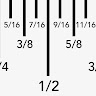## Mathematics# Direct and Inverse Proportionality## 11 questions# Introducing newPaper mode

No student devices needed. Know more

See Preview
• 1. Multiple-choice
2 minutes
1 pt

y is directly proportional to x, and y = 24 when x = 4.

What is the value of y when x = 3?

18

20

23

43

• 2. Multiple-choice
2 minutes
1 pt

The circumference (C cm) of a circle is directly proportional to its diameter (d cm).

The circumference of a circle of diameter 3.5 cm is 11 cm

What is the circumference of a circle of diameter 4.2 cm?

9.17 cm

11.7 cm

13.2 cm

14 cm

• 3. Multiple-choice
45 seconds
1 pt

P is directly proportional to T.

Which of the following could be the graph connecting T and P?